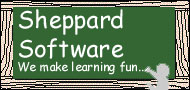Home | Software | Web Games | Quizzes | Free for Teachers | Patents | E-mail Us | Links
 Games One and Two: License Plate Math Here are daily games you can play on the road as well as on the computer!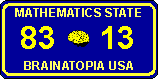Rules For License Plate Math (Game One)

Taking each of the two numbers on the license plate, determine if they match according to these four rules:

1) They match if the product of one of the numbers times an integer equals the other number, e.g.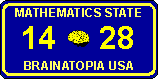28 equals 2 times 14.

2) They match if the sum of the digits of one number equals the other number, e.g.Look at the second number 28. It's tens digit is 2 and its units digit is 8. The sum of its two digits is 8 + 2 = 10 which is equal to the first number.

3) They match if the product of the digits of one number equals the other number, e.g.Look at the second number 28. It's tens digit is 2 and its units digit is 8. The product of its two digits is 8 x 2 = 16 which is equal to the first number.

4) They match if the sum, difference,product, or division of the digits of one number equals the sum, difference, product, or division of the digits of the other number, e.g.: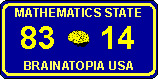The difference of the digits of the first number 83 equals the sum of the digits of the second number 14. 8 - 3 = 1 + 4

Note:
The difference of digits is always the larger digit minus the smaller digit. The difference is always positive or zero.
Also, The division of digits is always the larger digit divided the smaller one. The numbers must also divide evenly. Therefore for the number 14 the division of digits = 4/1 = 4. For the number 83 the division operation is invalid because 3 doesn't divide evenly into 8.

To sum up:
The two numbers on the license plate match if adding, subtracting, multiplying, or dividing the digits of one of the numbers is equal to adding, subtracting, multiplying or dividing the digits of the other number. Also they match if the product or sum of the digits of one number equals the other number. Finally they also match if the product of one of the numbers by an integer equals the other number.

Try some examples: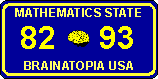Do you see the answer? Hint - subtract the digits of the first number: 8 - 2 = 6

scroll

8 - 2 = 9 - 3
The difference of the digits of the first number equals the difference of the digits of the second number.

Try another puzzle:Hint - take the product of the digits of the first number 7 x 0 = 0

scroll

7 x 0 = 2 - 2
The product of the digits of the first number equals the difference of the digits of the second number.

Try another puzzle: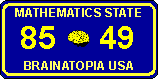Hint - try adding the digits of the first number.

scroll

8 + 5 = 4 + 9
The sum of the digits of the first number equals the sum of the digits of the second number.

More Examples: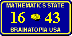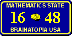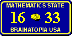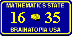6 + 1 = 4 + 3 The sum of digits of the first number equals the sum of digits of the second number. 16 x 3 = 48 The product of the first number times an integer equals the second number. 1 x 6 = 3 + 3 The product of digits of the first number equals the sum of digits of the second number. No Match! Not every plate matches.

The rules for matching are the same as Game One, but you must find the license plate with two or more matches!

Note: To count as another match, the match must be to another number. Thus the following plate plate only counts as one match 1 x 6 = 3 + 3 = 6 even though 6/1 = 3 + 3 = 6 is also a match, but to the same matching number 6. See examples of two valid matches below. Note that additional valid matches are always to a different matching number: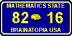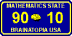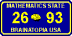14 = 2 x 7 and 1 + 4 = 7 - 2 8 x 2 = 16 and 8 - 2 = 1 x 6 90 = 10 x 9 and 9 x 0 = 1 x 0 17 x 3 = 51 and 7 - 1 = 5 + 1 2 x 6 = 9 + 3 and 6/2 = 9/3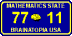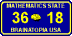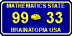7 - 7 = 1 - 1 7/7 = 1/1 1 + 4 = 7 - 2 3 x 6 = 18 3 + 6 = 1 + 8 36 = 18 x 2 99 = 33 x 3 9 - 9 = 3 - 3 9/9 = 3/3 66 = 11 x 6 6 - 6 = 1- 1 6/6 = 1/1 63 = 21 x 3 6 - 3 = 2 + 1 6/3 = 2/1